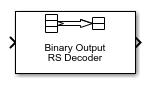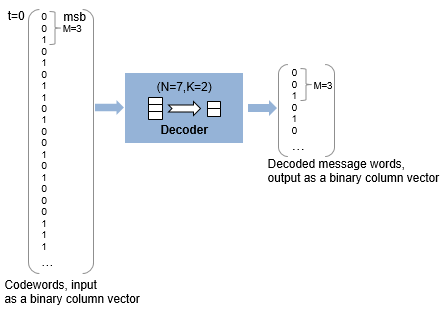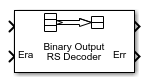# Binary-Output RS Decoder

Decode Reed-Solomon code to recover binary vector data

• Library:
• Communications Toolbox / Error Detection and Correction / Block

•## Description

The Binary-Output RS Decoder block recovers a binary message vector from a binary Reed-Solomon codeword vector. For proper decoding, the parameter values in this block must match parameter values in the corresponding Binary-Input RS Encoder block.

The symbols for the code are binary sequences of length M, corresponding to elements of the Galois field GF(2M). The first bit in each symbol is the most significant bit.

This figure shows the decoder input-output word length for codeword length N=7 and message word length K=2. Since N=2M–1, when N=7, the symbol length, M=3.Each input codeword is a binary vector of length 21 that represents 7 three-bit integers. Each corresponding output message word is a binary vector of length 6, that represents 2 three-bit integers. For more information, see Input and Output Signal Length in RS Blocks.

This icon shows all ports, including optional ones:## Ports

### Input

expand all

Reed-Solomon codeword in bits, specified as an (NC×(NK + SPM)-by-1 binary column vector. NC is the number of codewords, N is the Codeword length N (symbols), K is the Message length K (symbols), S is the Shortened message length S (symbols), P is the number of punctures per codeword, and M is the number of bits per symbol.

For more information, see Input and Output Signal Length in RS Blocks.

Data Types: `single` | `double` | `int8` | `int16` | `int32` | `uint8` | `uint16` | `uint32` | `Boolean` | `ufix(1)`

Erasure vector in symbols, specified as an (NC×(NK + SP))-by-1 binary column vector. NC is the number of codewords, N is the Codeword length N (symbols), K is the Message length K (symbols), S is the Shortened message length S (symbols), P is the number of punctures per codeword, and M is the number of bits per symbol.

Erasure values of 1 correspond to erased bits in the same position in the codeword. Values of 0 correspond to bits that are not erased. For more information, see Puncturing and Erasures.

#### Dependencies

To enable this port, select Enable erasures input port.

Data Types: `double` | `Boolean`

### Output

expand all

Decoded message in bits, returned as one of the following:

• When there is no message shortening, a (NC×K×M)-by-1 binary column vector.

• When there is message shortening, a (NC×S×M)-by-1 binary column vector.

NC is the number of message words, K is the Message length K (symbols), M is the number of bits per symbol, and S is the Shortened message length S (symbols).

Note

The number of decoded message words equals the number of codewords.

For more information, see Input and Output Signal Length in RS Blocks.

Data Types: `single` | `double` | `int8` | `int16` | `int32` | `uint8` | `uint16` | `uint32` | `Boolean` | `ufix(1)`

Symbol decoding errors, returned as an integer vector with NC elements, where NC is the number of codewords. This port indicates the number of symbol errors detected during decoding of each codeword. A negative integer indicates that the block detected more errors than it could correct by using the specified coding scheme.

Note

An (N,K) Reed-Solomon code can correct up to `floor((N-K)/2)` symbol errors (not bit errors) in each codeword. When a received codeword contains more than (N-K)/2 symbol errors, a decoding failure occurs.

#### Dependencies

To enable this port, select Output number of corrected symbol errors.

Data Types: `double`

## Parameters

expand all

Codeword length in symbols, specified as an integer.

For more information, see Restrictions on M and Codeword Length N and Input and Output Signal Length in RS Blocks.

Message word length in symbols, specified as an integer in the range [1, N–2], where N is the codeword length.

Shortened message word length in symbols, specified as an integer, such that SK. When Shortened message length S (symbols) < Message length K (symbols), the Reed-Solomon code is shortened.

You still specify N and K values for the full-length (N, K) code but the decoding is shortened to an (NK+S, S) code.

#### Dependencies

To enable this parameter, select .

Generator polynomial with values from 0 to 2M–1, in order of descending power, specified as one of the following:

• A polynomial character vector. For more information, see Representation of Polynomials in Communications Toolbox.

• An integer row vector that represents the coefficients of the generator polynomial in order of descending power.

• An integer Galois row vector that represents the coefficients of the generator polynomial in order of descending power.

Each coefficient is an element of the Galois field defined by the primitive polynomial. For more information, see Specify the Generator Polynomial.

Example: `[1 3 1 2 3]`, which is equivalent to `rsgenpoly(7,3)`

#### Dependencies

To enable this parameter, select .

Primitive polynomial in order of descending power. This polynomial is of order M and defines the finite Galois field GF(2M) corresponding to the integers that form message words and codewords. Specify the primitive polynomial as one of the following:

Example: `'X^3 + X + 1'`, which is the primitive polynomial used for a (7,3) code, ```ppoly = primpoly(3,'nodisplay'); int2bit(ppoly,ceil(log2(max(ppoly))))'```

#### Dependencies

To enable this parameter, select .

Puncture vector, specified as an (NK)-by-1 binary column vector. Element indices with `1`s represent data symbol indices that pass through the block unaltered. Element indices with `0`s represent data symbol indices that get punctured, or removed, from the data stream. For more information, see Puncturing and Erasures.

#### Dependencies

To enable this parameter, select .

Selecting this check box enables the erasures port, Era. For more information, see Puncturing and Erasures.

Selecting this check box enables an additional output port, Err, which indicates the number of symbol errors the block corrected in the input codeword.

Output type of the block, specified as ```Same as input```, `boolean`, or `double`.

## Block Characteristics

 Data Types `Boolean` | `double` | `fixed point[a]` | `integer` | `single` Multidimensional Signals `no` Variable-Size Signals `no` [a] ufix(1) only.

expand all

## Algorithms

This block uses the Berlekamp-Massey decoding algorithm. For information about this algorithm, see Algorithms for BCH and RS Errors-only Decoding.

 Wicker, Stephen B. Error Control Systems for Digital Communication and Storage. Upper Saddle River, NJ: Prentice Hall, 1995.

 Berlekamp, Elwyn R. Algebraic Coding Theory. New York: McGraw-Hill, 1968.

 Clark, George C., Jr., and J. Bibb Cain. Error-Correction Coding for Digital Communications. New York: Plenum Press, 1981.